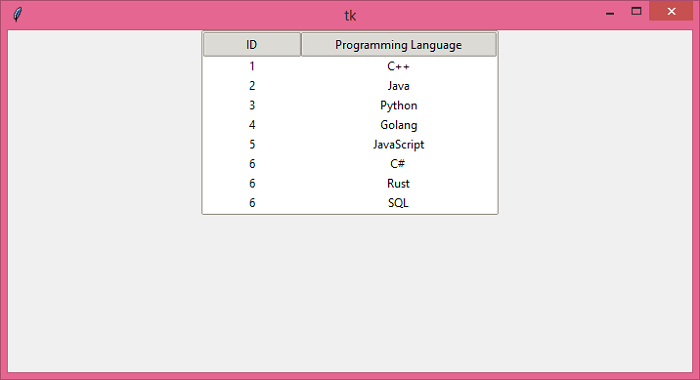# How to change ttk.Treeview column width and weight in Python 3.3?

TkinterPythonGUI-Programming

To display a large set of data in a Tkinter application, we can use the Treeview widget. Generally, we represent data through tables that contain a set of rows and columns. We can add the data in the form of a table with the help of the Treeview widget.

To configure the column width of the Treeview widget, we can use the width and stretch property. It sets the width of the Treeview widget column with the given value.

## Example

In this example, we have created a table that contains a list of programming languages. The width of columns ‘ID’ and ‘Programming Language’ is set to their content. Further, we can give a value to set the width of the columns.

# Import the required libraries
from tkinter import *
from tkinter import ttk

# Create an instance of tkinter frame
win=Tk()

# Set the size of the tkinter window
win.geometry("700x350")

# Create an instance of Style widget
style=ttk.Style()
style.theme_use('clam')

tree.column("# 1",anchor=CENTER, stretch=NO, width=100)
tree.column("# 2", anchor=CENTER, stretch=NO)

# Insert the data in Treeview widget
tree.insert('', 'end',text="1",values=('1','C++'))
tree.insert('', 'end',text="2",values=('2', 'Java'))
tree.insert('', 'end',text="3",values=('3', 'Python'))
tree.insert('', 'end',text="4",values=('4', 'Golang'))
tree.insert('', 'end',text="5",values=('5', 'JavaScript'))
tree.insert('', 'end',text="6",values=('6', 'C# '))
tree.insert('', 'end',text="7",values=('6', 'Rust'))
tree.insert('', 'end',text="8",values=('6', 'SQL'))

tree.pack()
win.mainloop()

## Output

Run the above code to display a Table that contains a list of programming languages and Index.